Learn US

# How to perform operations related to algebraic thinking?

386 views

 1 Introduction 2 What is Algebraic Thinking 3 Algebraic thinking for Grade 2 4 Examples 5 Operations and Algebraic Thinking Grade 2 Worksheet 6 Summary 7 About Cuemath 8 FAQs 9 External References

7 January 2021

## Introduction

Grade 2 is the grade where children will move from using base 10 numbers to two-digit numbers and end up using the arithmetic operations such as addition and subtraction till 100.

At the end of Grade 2, students will be able to use mental strategies and can effectively solve the addition and subtraction of 2-digit numbers fluently. Their algebraic thinking will improve and be ready to face more arithmetic challenges in the upcoming grades.

If you ever want to read it again as many times as you want, here is a downloadable PDF to explore more.

## What is Algebraic Thinking

Algebraic thinking is the way of identifying the patterns, understanding the changes based on their relationships. It is the ability to recognize the symbols used in the statement and analyze the change algebraically.

It’s the knowledge to identify abstract symbolism and the capability of applying the acquired algebraic skills in solving various problems. Algebraic thinking can be introduced as early when the student is introduced to mathematics.

## Algebraic thinking for Grade 2

In Grade 1, a child will learn the base ten method and use the same for identifying the numbers in doubles; this lays the foundation of them to identify whether the number is odd or even using them as pairs.

Addition and subtraction are the first steps of arithmetic operations introduced to a child which helps them to solve some basic and common one to two-line word problems involving algebraic thinking by comparing the numbers, sorting it out and adding together.

## Examples

 Introducing the addition and Subtraction: Algebraic thinking based on doubles: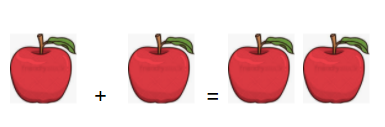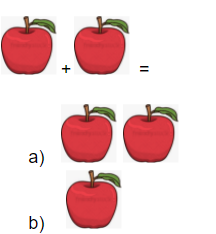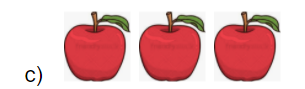## Operations and Algebraic Thinking Grade 2 Worksheet

Worksheet no: 1

 Colour, Pair them and circle whether they are odd or even: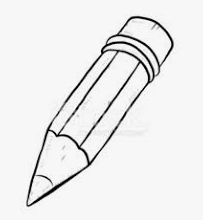a)   Odd b) Evena)   Odd b) Even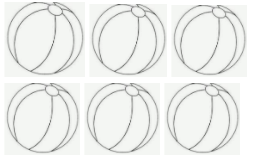a)   Odd b) Even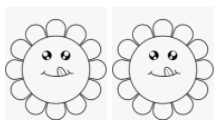a)   Odd b)   Even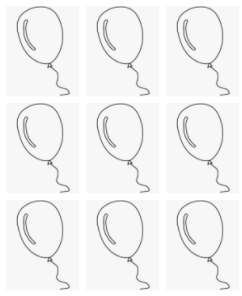a)   Odd b)   Even

Worksheet 2:

 Colouring and Algebraic thinking on Addition and Subtraction Circle the correct answer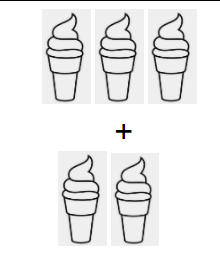a)   8 b)   5 c)   3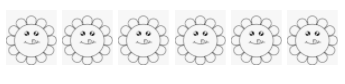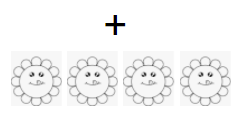a) 10 b)   6 c)    4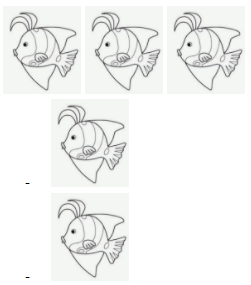a) 1 b)  3 c)  8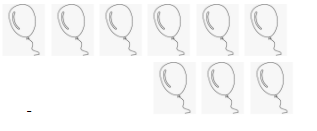a) 3 b)  0 c)  5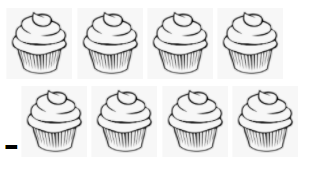a) 9 b) 0

Worksheet - 3

 Word Problems on Algebraic thinking: 1. Sarah had 3 pencils in her pencil box her mom gave her another 2 pencils for her exams. How many pencils did Sarah have in total? a)    5 b)    8 c)    4 2.  Rama had 5 chocolates he gave 2 of  them to his baby brother. How many chocolates are now left with Rama? a) 0 b)  10 c)  7 3. Peter gave 2 pens to his friend Paul; he then gave 5 pens to Nancy, now Peter is left with 3 pens. How many pens did Peter have in total? a)   8 b)  10 c)   4

## Summary

When the child moves from Base ten’s comfort zone to the next step of learning two digit to three digit numbers; he is introduced to the main two arithmetic operations, Addition and Subtraction apart from the doubles he learnt in the previous grade.

The learning doesn’t stop there; his/her problem analyzing and sorting out skills helps him/her to widen the knowledge of algebraic thinking that he implements in everyday life.

-Written by Santhalakshmi Panchacharam, Cuemath Teacher

Cuemath, a student-friendly mathematics platform, conducts regular Online Live Classes for academics and skill-development and their Mental Math App, on both iOS and Android, is a one-stop solution for kids to develop multiple skills. Understand the Cuemath Fee structure and sign up for a free trial.

## What is algebraic thinking?

Algebraic thinking is recognizing and analyzing the problem based on their patterns and understanding how things change. Algebraic thinking should be introduced to the child as soon as they begin their way to learn mathematics. It is imperative in an early childhood education as it paves the course of different perceptions for a child to look into a problem.

## How to learn algebraic thinking?

Algebraic thinking is something that can be developed using their basic instincts.
One can develop their algebraic thinking by

•  using different strategies and approaches to solve one problem
• Using patterns, symbols, drawing etc.,
• Inductive and deductive reasoning
• Analyzing the problem and implementing them to various other problems to find out whether the approach is suitable for all the given calculations